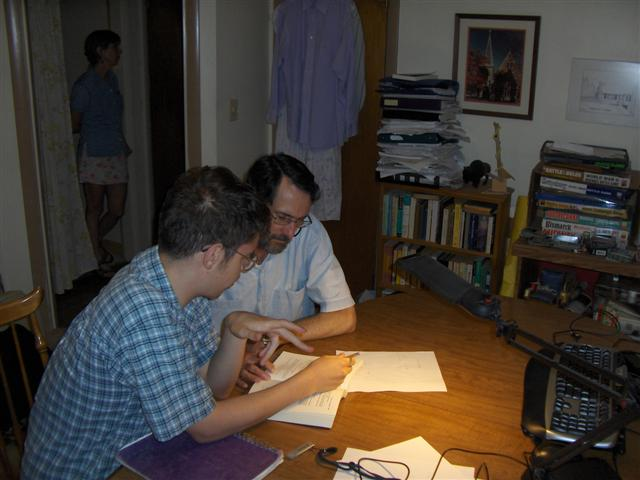## No Longer Tutoring

With the appearance of covid, and my advancing age and precarious health, I have brought my tutoring to an end. I leave this information up because the topics have been interesting and useful to readers (thanks for the notes saying so, and for the suggestions for improvement!). But I am no longer in the tutor business. I apologize for misleading anyone, and best wishes for finding the help you seek.

## Math and Science

### Mathematics

Is your elementary-school child having trouble with fractions? Your high-schooler can't quite get the quadratic formula? Maybe your college student is struggling with the Intermediate Value Theorem in his calculus class. Or perhaps you have a bright middle-schooler whose classes are too elementary and who wants to know what real mathematics is all about, the math that goes beyond just plugging three numbers into the Pythagorean equation to see whether they can form the sides of a right triangle. I've tutored local students in all those areas of mathematics.

Here's an example of how I work with people needing help with basic arithmetic. More advanced students wanting to explore the world of real mathematics have to understand what it means to prove things, so I might start with this introduction to proof. And then for further work we could explore a problem in graph theory or infinite sets for our topic.

### Science

In chemistry, the students might be asked to find the enthalpy change of a chemical reaction where the amounts of the reactants and the temperature change are given. This involves balancing the chemical equation, finding the limiting reactant, determining the energy released or absorbed from specific heat data, and finally scaling the energy to what would be seen due to the limiting reactant. Want an example?

A common high-school physics problem in ballistics will involve the equations connecting time, distance, and acceleration. The student will be given one quantity and asked to find another, which will require working through the several equations to get from the given quantity to the one asked for. Might be more interesting, though; maybe we can figure out how fast we can take a turn in a car without skidding.

Remember Deflategate? What can high-school physics tell us about the credibility of the Patriots' defense? Read the analysis here.